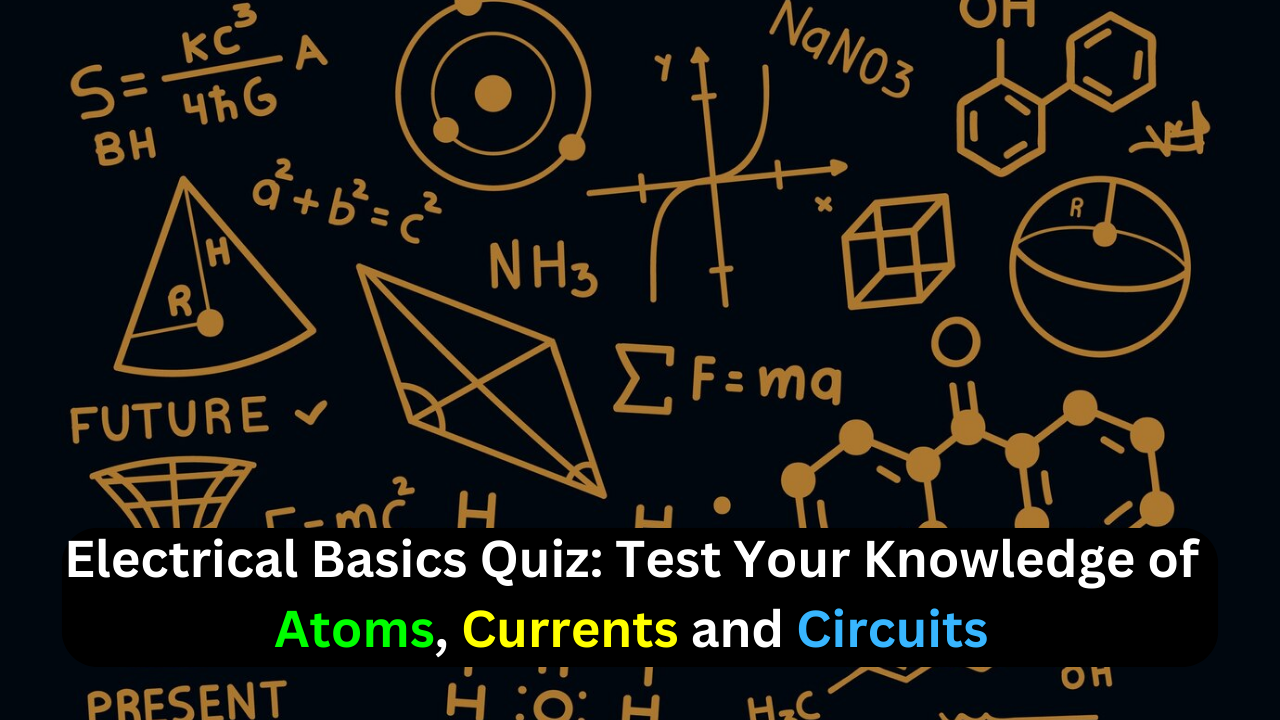Thursday, October 26

## Electrical Basics Quiz: Test Your Knowledge of Atoms, Current, and Circuits### How many electrons does a neutral atom with an atomic number of three have?

(a) 1
(b) 3
(c) none
(d) depends on the type of atom

(a) shells
(b) nuclei
(c) waves
(d) valences

### What are materials in which current cannot be established called?

(a) filters
(b) conductors
(c) insulators
(d) semiconductors

### When placed close together, a positively charged material and a negatively charged material will

(a) repel
(b) become neutral
(c) attract
(d) exchange charges

### What is another term for the potential difference?

(a) energy
(b) voltage
(c) distance of an electron from the nucleus
(d) charge

(a) watt
(b) coulomb
(c) joule
(d) volt

### Which of the following is not a type of energy source?

(a) battery
(b) solar cell
(c) generator
(d) potentiometer

### What is the byproduct of a hydrogen fuel cell?

(a) oxygen
(b) carbon dioxide
(c) hydrochloric acid
(d) water

### What is generally not a possible condition in an electric circuit?

(a) voltage and no current
(b) current and no voltage
(c) voltage and current
(d) no voltage and no current
Answer: (d) no voltage and no current

### How is electrical current defined?

(a) free electrons
(b) the rate of flow of free electrons
(c) the energy required to move electrons
(d) the charge on free electrons
Answer: (b) the rate of flow of free electrons

### When is there no current in a circuit?

(a) a series switch is closed
(b) a series switch is open
(c) there is no source voltage
(d) both (a) and (c)
(e) both (b) and (c)
Answer: (e) both (b) and (c)

### What is the primary purpose of a resistor?

(a) increase current
(b) limit current
(c) produce heat
(d) resist current change

### What are potentiometers and rheostats types of?

(a) voltage sources
(b) variable resistors
(c) fixed resistors
(d) circuit breakers

### The current in a given circuit is not to exceed 22 A. Which value of fuse is best?

(a) 10 A
(b) 25 A
(c) 20 A
(d) a fuse is not necessary